# Comparative Study on the Performance of Flat Tube Type and Wasp-Waisted Tube Type Radiators

Comparative Study on the Performance of Flat Tube Type and Wasp-Waisted Tube Type Radiators

Ying HuangLunjun Chen Meijun Li Bin Zhang Linna Zhang

College of mechanical engineering, Gui Zhou University, Huaxi District, Guiyang, 550025, China

Yonghong Radiator Company, Xiaohe District, Guiyang, 550009, China

Corresponding Author Email:
1954174359@qq.com
Page:
647-652
|
DOI:
https://doi.org/10.18280/ijht.340414
|
Accepted:
|
Published:
31 December 2016
| Citation

OPEN ACCESS

Abstract:

Keywords:

Car radiator, Flat tube type, Heat dissipation performance, Wasp-waisted type.

1. Introduction

The improvements in the performance of radiator have attracted many researchers for a long time as they are of great technical, economical, and ecological importance. The main subject to design radiator is how to enhance the heat transfer process, so that its integral performance may be improved to meet the demand of energy saving and low cost with the volume as small as possible and the weight as light as possible.

## 1.png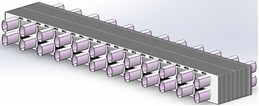Figure 1. Sketch map of flat tube type assembled radiator core body

At present, most assembled radiators are equipped with a flat radiating tube. Compared with the traditional circular tube, the flat tube has a larger heat transfer surface area, and better heat transfer efficiency. Besides, the flat tube needed to transfer the same amount of heat is much lighter in weight and smaller in size than the circular tube.

Although numerous studies have been conducted on the characteristics of flow and heat transfer in round, elliptical, and flat tubes, studies on new types of tubes are limited, especially on special section tubes, recommended that further detailed studies via numerical simulations and/or experimental investigations should be carried out.

3. Numerical Simulation Analysis of Flat Tube Type and Wasp-Waisted Tube Type Assembled Radiators

As an indispensable part of the radiator, the radiating tube has a certain influence on the distribution of the flow field and the heat transfer efficiency. Through a numerical study of circular and oval tubes of approximately equal diameter and fin area, Li Zhang et al.  discovered that the oval tube has a better heat dissipation effect due to its larger surface area. Xiuqin Ma et al.  conducted a simulation analysis of how different types of radiating tube affect heat dissipation performance, but the simulation failed to pass experimental verification. Foreign scholars have also conducted a lot of research on the radiating pipe distribution, airside coupled heat transfer, and the establishment of a mathematical model of louvered fin radiator [8, 9, 10]. Xiaoli Yu et al. of Zhejiang University applied CFD simulation technology to the flow field, pressure loss, and heat dissipation characteristics in radiator components, and verified the simulation in a wind tunnel . In this paper, the author compares the heat dissipation performance of flat tube type and wasp-waisted tube type assembled radiators, which have the same structure parameters (base tube equivalent diameter, fin thickness, and fin surface area), under the same conditions.

3.1 Theoretical basis

Flat radiating tubes are usually made by pressing a circular tube with the same circumference. See Figure 2. The wasp-waisted radiating tube, however, symbolizes a breakthrough in structural design: maximizing outer circumference and reducing the equivalent diameter without sacrificing the flow area. Besides, the “wasp-waist” increases the area of the radiating fin. Compared with the previous assembled products, the wasp-waisted tube features a much smaller tube spacing, more radiating units, and more compact core structure. See Figure 3.

## 2.png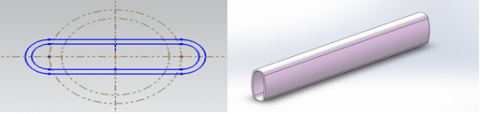Figure 2. Sketch map of flat radiating tube

## 3.pngFigure 3. Sketch map of wasp-waisted radiating tube

The radiator has a complex flow and heat transfer process. The fluid flow and heat transfer meet the laws of conservation of mass, energy, and momentum [12, 13].

Conservation of mass equation: The mass increase in fluid microelement per unit time is equal to the net mass which flows into the microelement in the same time interval.

$\frac{\partial \rho}{\partial t}+\frac{\partial(\rho u)}{\partial x}+\frac{\partial(\rho v)}{\partial y}+\frac{\partial(\rho w)}{\partial z}=0$(1)

Conservation of momentum equation: the change rate of the fluid in the microelement with respect to time equals the sum of various external forces acting on the microelement.

$\frac{\partial(\rho u)}{\partial t}+d i v(p u u)=\operatorname{div}(\mu g r a d u)-\frac{\partial \rho}{\partial x}+S_{U}$(2)

$\frac{\partial(\rho v)}{\partial t}+\operatorname{div}(p v u)=\operatorname{div}(\mu g r a d v)-\frac{\partial \rho}{\partial x}+S_{v}$(3)

$\frac{\partial(\rho w)}{\partial t}+\operatorname{div}(p w u)=\operatorname{div}(\mu g r a d w)-\frac{\partial \rho}{\partial x}+S w$(4)

Conservation of energy equation: The increase rate of the energy in the microelement is equal to the net heat flow into the microelement plus the work done by the body and surface forces on the microelement. The conservation of energy equation with temperature T as the variable is:

$\frac{\partial(\rho T)}{\partial t}+\operatorname{div}(p u T)=\operatorname{div}\left(\frac{k}{c_{p}} g r a d T\right)-\frac{\partial \rho}{\partial x}+S_{T}$(5)

Since a radiator involves a large number of heat transfer tubes and a complex fin structure, the computing power of existing computers is not enough to simulate the fluid flow in the radiator. Thus, the radiator model must be simplified to a certain extent.

3.2 Simplification and establishment of geometric model

## 4.png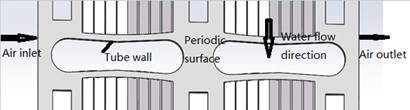Figure 4. Geometric model of wasp-waisted tube type radiator

3.3 Basic assumptions and boundary condition settings

With water as the cooling liquid and without considering the structure of the radiating fin, the heat pipe and radiating fin are modeled as one body to eliminate thermal resistance. In addition, the author assumes that the air is incompressible, the physical parameters of the air are constant, the air flow is steady, the velocity direction of the air is vertical to the windward side, and the air is evenly distributed in the windward side, and ignores the impact of air gravity. To ensure the stability of the flow and the reliability of the physical model, the calculation area is extended in all directions rather than just the actual area.

The specific boundary conditions are defined as follows:

1) The air inlet is set as the boundary of velocity inlet y, and the air inlet temperature is set to 293 K.

2) The air outlet is set as the boundary of the pressure outlet.

3) Inlet water temperature: 358 K, water flow: 100 L / min.

4) Wind speed: 2 m/s, 4 m/s, 6 m/s, 8 m/s, and 10 m/s.

5) The fluid is evenly distributed in the flow channel and is fully developed.

6) The upper and lower planes are set as the periodic boundaries.

7) The symmetry plane is set as the symmetrical boundary.

8) The interface between the solid and the fluid regions is the coupled heat transfer surface.

3.4 Results and analysis

The simulation results of the wasp-waisted tube and the flat tube are described at the air inlet temperature of 293 K, wind speed of 4 m/s, inlet water temperature of 358 K, and water flow rate of 100 L/min. As shown in Figure 5, the air flow rate near the inlet in both figures is increased as the heat pipe reduces the air flow area. For both tubes, the regions with the highest velocity appear in the region near the air inlet and outlet where the air flow channel is much narrower than other places. As the outside wall of the flat tube is a plane surface, the air flows at a uniform velocity, but local turbulence occurs after the air flows around the radiating tube. In contrast, the wasp-waisted tube is a special tube with concave walls and a longer outer circumference than the flat tube. The special shape increases the area of turbulence. The air flow throughout the air channel of the wasp-waisted tube is much more chaotic than that of the flat tube. As shown in Figure 6, the cooling air enters from the left side of the model and keeps transferring heat by convection with the surface of the louver fins, leading to a decrease in the temperature of the fin surface and a gradual increase in the cooling air temperature. As the heat transfer progresses, the temperature difference between the fin surface and the cooling gas becomes smaller and smaller. From the length of the red region, it can be inferred that the heat dissipation efficiency of the wasp-waisted tube is higher than that of the flat tube. What’s more, obvious eddy currents are formed between the two tubes in each figure. There are more eddy currents near the wasp-waisted tube than the flat tube. Under the same conditions, more eddy currents can carry away more heat. For the flat tube, the outlet wind temperature is 350.73 K, and the wind side pressure drop is 161 Pa; for the wasp-waisted tube, the outlet wind temperature is 351.265 K, and the wind side pressure drop is 155 Pa. Thus, the wasp-waisted tube has a higher outlet wind temperature and lower wind resistance than the flat tube, indicating that wasp-waisted tube type radiators dissipate more heat at the wind side than flat tube type radiators. However, the wasp-waisted tube has a higher water resistance because of its irregular shape.

## 5a.png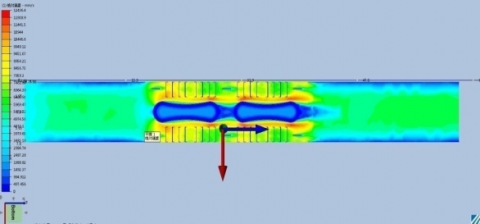(a) Wind side velocity distribution of wasp-waisted tube

## 5b.png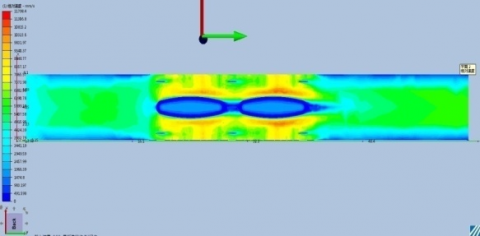(b) Wind side velocity distribution of flat tube

Figure 5. Wind side velocity distribution

## 6a.png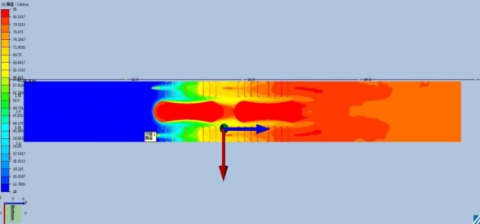(a) Wind side temperature distribution of wasp-waisted tube

## 6b.png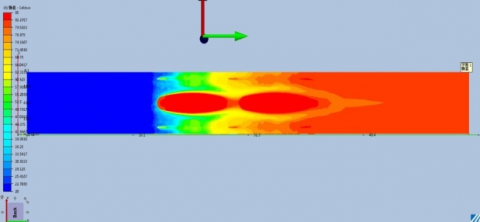(b) Wind side temperature distribution of flat tube

Figure 6. Wind side temperature distribution

3.5 Comprehensive performance evaluation

As stated above, the flat tube has a lower heat transfer coefficient and a lower water resistance than the wasp-waisted tube. Thus, an increase of the heat transfer coefficient inevitably drives up the pressure drop, which calls for a standard to comprehensively evaluate the performance of radiating tubes. Therefore, the comprehensive evaluation factor of radiating tube performance as the evaluation standard is introduced. Reflecting the energy transferred with the same amount of pump power, the factor gives comprehensive consideration to the influence of heat transfer and pressure drop. The greater the factor is, the better the comprehensive performance. Whereas the comprehensive performance evaluation factor of the wasp-waisted tube is always higher than that of the flat tube, it is proved that a wasp-waist structure can improve the comprehensive performance of radiating pipes.

4. Experimental Verification of Heat Dissipation Performance of Flat Tube Type and Wasp-Waisted Tube Type Assembled Radiators

As an internationally used radiator test method, wind tunnel test can accurately measure such performance parameters of radiator as inlet/outlet temperature difference, inlet/outlet pressure drop and heat dissipating capacity by adjusting the cooling air flow rate, water flow, etc. The experiment uses artificial air flow channel to provide cooling air to the radiators. Major experiment equipment include air duct, air duct circulating water circuit, circulating water heating device, wind speed measuring instrument, temperature sensor, pressure sensor, mass flow meter and fan. The air duct creates the flow channel, and the fan provides power. Under the combined action of the air duct and the fan, the air flows through the radiator. In order to compare the performance difference between the two types of radiators, the author conducts a wind tunnel test for each, and draws charts to compare their heat dissipating capacity, wind resistance and water resistance. Figure 7 illustrates the test site.

## 7.png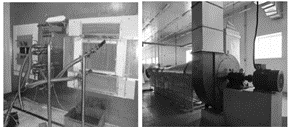Figure 7. Wind tunnel test rig

The experiment is carried out at room temperature. The ambient temperature is adjusted to 293 K, and the inlet air temperature fluctuation does not exceed ±5 °C. Besides, no obstacle exists within the distance of three times the air duct diameter from the inlet of the air duct. Five wind speed operating points are selected, namely 2 m/s, 4 m/s, 6 m/s, 8m/s, and 10 m/s. Figure 8 demonstrates the heat dissipating capacity of the two types of radiators under different wind speeds. As shown in the figure, the two types of radiators have a similar heat radiating capacity under low wind speed. As the wind speed increases, the heat radiating capacity of the wasp-waisted tube increases as well because the tube generates more eddy currents than the flat tube. The trend echoes the results of numerical simulation.

## 8.png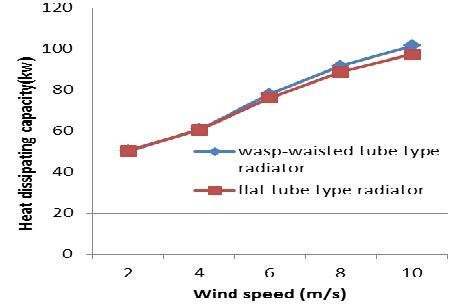Figure 8. Heat dissipating capacity of wasp-waisted tube type radiator and flat tube type radiator

As shown in Figure 9, the wind resistance of both types of radiators increases along with the wind speed. The wasp-waisted radiating tube has a lower wind resistance thanks to the larger fin area because the design maximizes outer circumference and reduces the equivalent diameter. Three water flow operating points are selected, namely 60 L/min, 87L/min, and 100 L/min. As shown in Figure 10, the wasp-waisted tube has a larger water resistance. With the aid of the comprehensive performance evaluation factor, it is found that the wasp-waisted tube type radiator has a better heat dissipation performance than that of the flat tube type radiator. The finding agrees with the results of numerical simulation.

## 9.png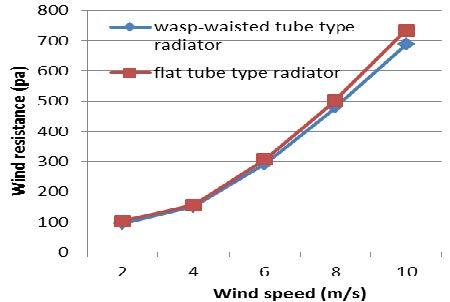Figure 9. Wind resistance of wasp-waisted tube type radiator and flat tube type radiator

## 10.png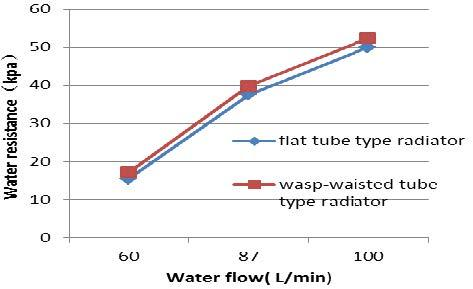Figure 10. Water resistance of wasp-waisted tube type radiator and flat tube type radiator

5. Conclusion and Outlook

Acknowledgment

This study was supported by Project of Guizhou Province Science and Technology Program (20146004).

Nomenclature

t         time

U       the weight of the velocity vectors in the x direction

V       the weight of the velocity vectors in the y direction

W      the weight of the velocity vectors in the z direction

P        the pressure on the fluid

Div    the divergence of mathematical operator

Su      the generalized source terms of the conservation of momentum equation

Sv      the generalized source terms of the conservation of momentum equation

Sw      the generalized source terms of the conservation of momentum equation

C P      specific heat, J. kg-1. K-1

T         temperature

k         thermal conductivity, W. m-1. K-1

ST           the internal heat source

Greek symbols

ρ          Density, kg. m-3

μ          Dynamic viscosity, kg. m-1. S-1

References

 S. H. Lee, N. Hur, S. Kang, “An efficient method to predict the heat transfer performance of a louver fin radiator in an automotive power system,” J Mech Sci Technol, vol. 28, no. 1, pp. 145–155, Jan., 2014. DOI: 10.1007/s12206-013-0951-8.

 D. Antonijevic, “An engineering procedure for air side performance evaluation of flat tube heat exchangers with louvered fins,” Heat Mass Transfer, vol. 49, no. 1, pp. 117-127, Jan., 2013. DOI: 10.1007/s00231-012-1073-z.

 Q. J. Guo, X. N. Qi, Z. Wei, et al, “3D numerical simulation and analysis of refrigeration performance of the small diameter vortex tube,”  Int J Heat & Tech, vol. 34, no. 3, pp. 513–520, Sep., 2016. DOI: 10.18280/ijht.340324.

 S. E. Rafiee, M. M. Sadeghiazad, “Heat and mass transfer between cold and hot vortex cores inside ranque-hilsch vortex tube-optimization of hot tube length,” Int J Heat & Tech, vol. 34, no. 1, pp. 31-38, 2016. DOI: 10.18280/ijht.340105.

 S. A. E. Sayed Ahmed, O. M. Mesalhy, M. A. Abdelatief, “Flow and heat transfer enhancement in tube heat exchangers,” Heat Mass Transfer, vol. 51, no. 1, pp. 1607-1630, Nov., 2015. DOI: 10.1007/s00231-015-1669-1.

 L. Zhang, K.Yang, W. Liu, “Numerical study on flow and heat transfer in an elliptical and circular finned tube,” Journal of Engineering Thermophysics, vol. 30, no. 9, pp. 1571-1574, Sep.,  2009.

 X. Q. Ma, J. Zhao, M. Q. Zheng, et al, “Study on the effect of tube type on the heat transfer performance,” Machinery Design & Manufacture, no. 8, pp. 210-213, Aug., 2015.

 T. K. Kim, H. C. Kang, J. S. Lee, “A porosity model for flow resistance calculation of heat exchanger with louvered fins,” J Mech Sci Technol, vol. 30, no. 4, pp. 1943-1948, Apr., 2016. DOI: 10.1007/s12206-016-0353-9.

 C. Cuevas, D. Makaire, L. Dardenne, P. Ngendakumana, “Thermo-hydraulic characterization of a louvered fin and flat tube heat exchanger,” Exp Thermal Fluid Sci, vol. 35, pp.154-164, Jan., 2011. DOI: 10.1016/j.expthermflusci.2010.08.015.

 D. Taler, “Experimental determination of correlations for average heat transfer coefficients in heat exchangers on both fluid sides,” Heat Mass Transfer, vol. 49, no. 8, pp. 1125-1139, Aug., 2013. DOI: 10.1007/s00231-013-1148-5.

 Y. Q. Huang,  X. L.Yu, G. D. Lu, “Numerical simulation of slit fin cell’s flow and heat transfer,” Journal of Zhejiang University, vol. 42, no. 8, pp. 1462-1468, Aug., 2008.

 Z. G. Tong, Y. F. Wang, D. Chen, “Research on the structure optimization of automobile radiators,” Energy Research and Information, vol. 30, no. 2, pp.108-112, 2014. DOI: 10.13259/j.cnki.eri.2014.02.011.

 X. F. Pan, W. W. Cao, Q. L. Liu, et al, “Simulation research on car radiator fin,” Journal of Shanghai University of Engineering Science, vol. 28, no. 3, pp. 193-197, Sep., 2014.

 Q. L. Meng, M. D. Yin, Z. X. Zhu, “Numerical simulation study of the cold and hot side coupled heat dissipating of the vehicle radiator,” Machinery Design & Manufacture, no. 7, pp. 95-98, Jul., 2015.

 S. A. E. Sayed Ahmed, O. M. Mesalhy, M. A. Abdelatief, “Flow and heat transfer enhancement in tube heat exchangers,” Heat Mass Transfe, vol. 51, no. 11, pp. 1607-1630, Nov, 2015. DOI: 10.1007/s00231-015-1669-1.

 Y. Wu, J. Z. Guo, L. Hu, et al, “Simulation and test research for the multi-structure of automotive louvered fins heat exchangers,” Science Technology and Engineering, vol. 16, no. 11, pp. 59-64, Apr., 2016.